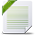# Decision Optimization

View Only

## OPL - Is there a way to check for memory leaks?

• #### 1.  OPL - Is there a way to check for memory leaks?

Posted Sat October 16, 2021 09:07 AM
Hello,

Consider the following code which solve a linear program 100 times:

main {
var NORUNS = 100;//Number of LPs to solve
var modelname="iterate.mod";
var source = new IloOplModelSource(modelname);
var def = new IloOplModelDefinition(source);
for(var probno = 1; probno <= NORUNS; probno++){
var cplex = new IloCplex();
var theModel = new IloOplModel(def, cplex);
var ifile = "input.dat";
var data = new IloOplDataSource(ifile);
theModel.generate();
if(cplex.solve()){
writeln(probno, ": ", cplex.getObjValue());
theModel.postProcess();
}
theModel.end();
cplex.end();
data.end();
}
def.end();
source.end();
};

iterate.mod:

int c[1..3] = ...;
int A[1..2][1..3] = ...;
int b[1..2] = ...;
dvar float+ X[j in 1..3];

maximize sum(j in 1..3) c[j]*X[j];

subject to{
forall(i in 1..2)
sum(j in 1..3) A[i][j]*X[j] <= b[i];
};

input.dat:

c = [2, 4, 7];

A = [
[1, 3, 2],
[2, 3, 3]
];

b = [4, 2];

(All these files are also attached with this post)

Different CPLEX objects are instantiated via function new: new IloCplex(); new IloOplModel(); etc.

The memory allocated to these are reclaimed in the code above: theModel.end(); cplex.end(); etc.

Suppose the latter .end()'s are not there in the code or are commented out, I would expect that the application would leak memory. Is there any way to "run" this application via some process that indicates where there is memory leak happening? I looked at the Profiler tab of the OPL IDE, but that only seems to indicate hotspots in the application and not whether the application is leaking memory or not.

In C/C++ (on linux), one could run the executable via valgrind to figure out memory leaks. Is there something equivalent for OPL models?

Thanks.

------------------------------
CPLEX User
------------------------------

Attachment(s)iterate.mod   204 B 1 versioninput.dat   81 B 1 versionopl.mod   642 B 1 version